log ⪢⪢
The pond makes it easier to move around the heavy logs they need to build the dams. Out of the water it's a struggle.
http://qindex.info/i.php?x=16396Logarithms Explained
In the same way that division is the inverse of multiplication a logarithm is just the inverse of exponentiation.
http://qindex.info/i.php?x=3738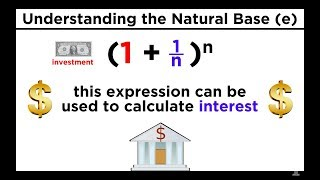42 Logarithms Part 2
Base Ten Logs, Natural Logs, and the Change-Of-Base Property
http://qindex.info/i.php?x=3639Change of base
Log base b of n. What power should I put in the exponent of b so b to that power will give me n.
http://qindex.info/i.php?x=3798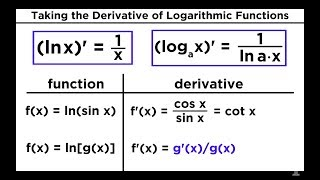09 Derivatives of Logarithmic and Exponential Functions
Derivatives of Logarithmic and Exponential Functions
http://qindex.info/i.php?x=3387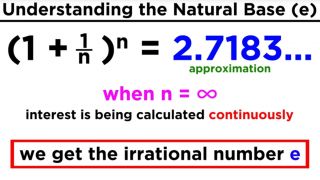42 Logarithms Part 2
Base Ten Logs, Natural Logs, and the Change-Of-Base Property
http://qindex.info/i.php?x=5041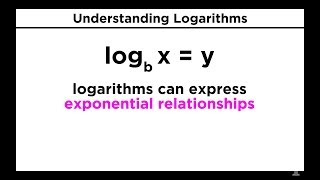41 Logarithms Part 1
When we say log base b of x equals y, we are saying b to the y equals x. With logs, the base of the log raised to the power of what's on the other side of the equal sign will equal the number that the log is operating on.
http://qindex.info/i.php?x=3638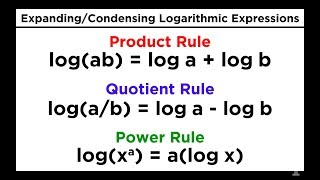43 Logarithms Part 3
Logarithms Part 3: Properties of Logs, Expanding Logarithmic Expressions
http://qindex.info/i.php?x=509243 Logarithms Part 3
Logarithms Part 3: Properties of Logs, Expanding Logarithmic Expressions
http://qindex.info/i.php?x=3640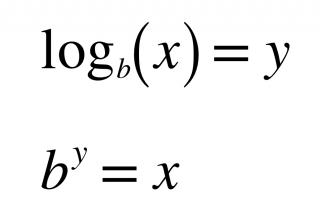○○○ logarithm ⪢⪢
So if you want to find log base b of x, you're asking "what power you have to raise b to in oder to get x?". #math
http://qindex.info/i.php?x=3784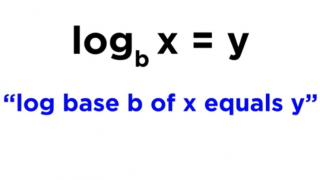○○○ logarithm ⪢⪢
When we say "log base b of x equals y", we are saying that b to the y equals x. ┆ x⍻b = y ➔ b˄y = x #math
http://qindex.info/i.php?x=6085
Logarithms Explained
What is the power that I should raise this base to in order to get this number?
http://qindex.info/i.php?x=9635
Logarithms Explained
Anytime you see log base b of some number n, you can think about it as asking the question "What power do I need to put in my exponent to get b to that power equal to this number n?"
http://qindex.info/i.php?x=9637
○○○ raise ⪢⪢
So if you want to find log base b of x, you're asking 'What power you have to _____ b to in oder to get x?'
http://qindex.info/i.php?x=9876
○○○ encroachment ⪢⪢
Due to logging and human encroachment, less than 25% of historical forest remains.
http://qindex.info/i.php?x=14864○○○ logarithm ⪢⪢
Or you can say "If I raise b to the power of y, I'm going to get x." #math
http://qindex.info/i.php?x=28242○○○ logarithm ⪢⪢
With logs, the base of the log raised to the power of what's on the other side of the equal sign will equal the number that the log is operating on. ┆ x⍻b = y ➔ b˄y = x #math
http://qindex.info/i.php?x=28240○○○ logarithm ⪢⪢
By definition, when we say "log base b of x equals y", that's the same thing as saying "b to the y equals x". #math
http://qindex.info/i.php?x=28241
-----